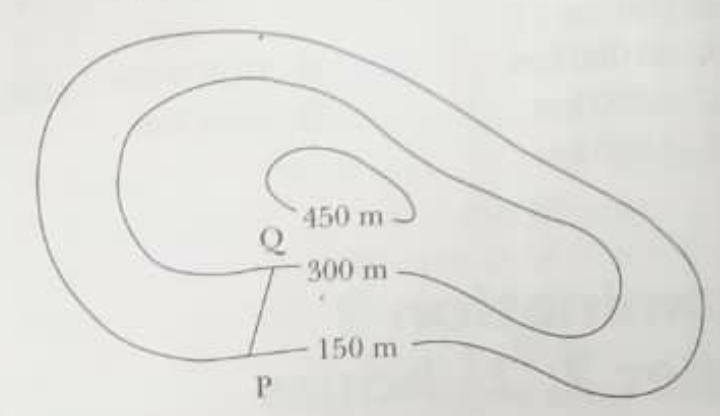mycollegehive
How to calculate the magnitude of PQ as well as the angle. Given the data and diagram below
math map0A map of a region is drawn to scale of 1cm to 100m. The diagram below is part of the map (reduced) showing contour linesPQ is a straight path with a constant gradient of 1 vertically in 6 horizontally. Calculate

1. The length of PQ as measured on the map
2. The actual length of the path PQ, correct to the nearest 10m
3. The angle of elevation of Q from P

43 viewsShareFollowUniversity of Benin Nigeria
13 May 2020### Related Tags

math

2 followers

993 questionsmap

0 followers

142 questions